## 8.8Venturi tube

A Venturi tube is a device used to measure the ﬂow rate of a ﬂuid in a pipe. The device exploits the Venturi eﬀect4, i.e. that pressure reduces when the ﬂuid ﬂows through a restriction.

The volumetric ﬂow rate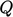is calculated according to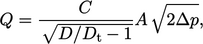(8.2)
where: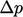is the decrease in kinematic pressure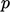between the Venturi inlet and throat;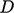and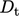are the inlet and throat diameter, respectively; the cross-sectional area is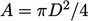; and,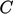is a discharge coeﬃcient. A coeﬃcient of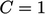satisﬁes the Bernoulli equation,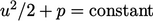, between the inlet and throat, where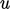is the ﬂuid speed.

For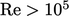,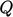is calculated accurately usingvalues between 0.97 and 1,5 but, for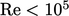, suitable values ofdecrease signiﬁcantly below 1 in order to account for pressure losses due to viscous forces.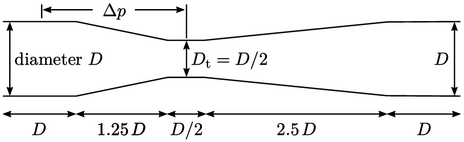A simulation was performed to calculate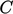for a Venturi tube, shown in the ﬁgure, with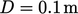and an inlet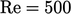. The inlet velocity was speciﬁed using the quadratic proﬁle in Sec. 8.7 with a mean cross-sectional speed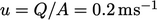, corresponding to a maximum speed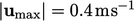.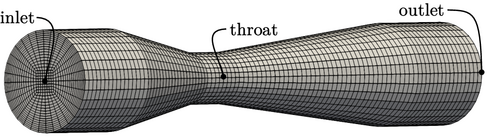The simulation used the steady-state algorithm in Sec. 5.12, with an incompressible ﬂuid with uniform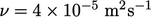. The mesh contained 57,600 cells, with a near-wall cell height of 1.5mm, which resolved the velocity proﬁles as shown below. The ﬂow recirculates near the wall downstream of the Venturi throat, causing inﬂow at the outlet boundary. Consequently, the total pressure and inlet-outlet-velocity boundary conditions, described in Sec. 4.7 and Sec. 4.15 respectively, were applied at the outlet to maintain stability.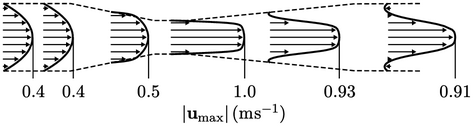The ﬂow was laminar so no turbulence modelling was required. The solution converged to within an absolute tolerance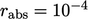, see Sec. 5.4 , in 292 iterations.

The pressure drop was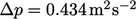between the centres of the inlet and throat sections, with a corresponding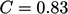.

4Giovanni Battista Venturi, Recherches expérimentales sur le principe de la communication latérale du mouvement dans les ﬂuides appliqué a l’explication de diﬀérens phénomènes hydrauliques, 1797.
5ISO 5167-4, Measurement of ﬂuid ﬂow by means of pressure diﬀerential devices inserted in circular cross-section conduits running full – part 4: venturi tubes, 2003.

Notes on CFD: General Principles - 8.8 Venturi tube